# Routh theorem

A theorem that enables one to determine, using a Routh scheme, the number of complex roots with positive real part of a polynomialwith real coefficients (in the regular case).

Writein the form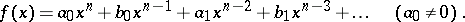The Routh scheme of this polynomial is defined to be the array of numbers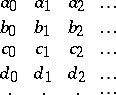In this scheme, the first two rows consist of the coefficients of, and every row from the third onwards is obtained from the previous two as follows: subtract from the first line that multiple of the second which makes the first entry equal to zero. By deleting this first zero, one obtains the described row. For example, in the third row,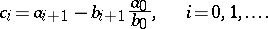The number of entries in the first row of the Routh scheme is equal to the integer part of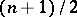, in the second, to that of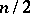, and in the-th (for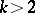), the number of entries is one less than in the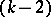-nd. The whole scheme containsrows. The case when the entries in the first column are all different from zero is called regular.

Routh's theorem: For a polynomial with real coefficients in the regular case, the number of roots lying in the right half-plane (that is, having positive real part) is equal to the number of changes of sign in the sequence of entries in the first column of the Routh scheme. In the regular case, the polynomial cannot have roots lying on the imaginary axis. Routh's criterion: Every root of a polynomialwith real coefficients has negative real part if and only if the entries in the first column of the Routh scheme are all non-zero and have the same sign. These theorems were established by E.J. Routh . Routh's scheme can also be used to determine the number of roots of a polynomial in the right half-plane in certain non-regular cases.

The construction of a Routh scheme is possible only for polynomials with given numerical coefficients. There is a more widely used method, in which the role of the Routh scheme is played by the Hurwitz matrix, and that of the first column by the sequence of principal minors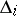,(see Routh–Hurwitz criterion; Minor). Then the analogue of Routh's theorem is the Routh–Hurwitz theorem: If the minorsare all non-zero, then the number of roots oflying in the right half-plane is equal to the number of changes of sign in the sequenceandhas no roots lying on the imaginary axis. Under certain extra conditions, this method can be used in case some of the minorsare equal to zero.